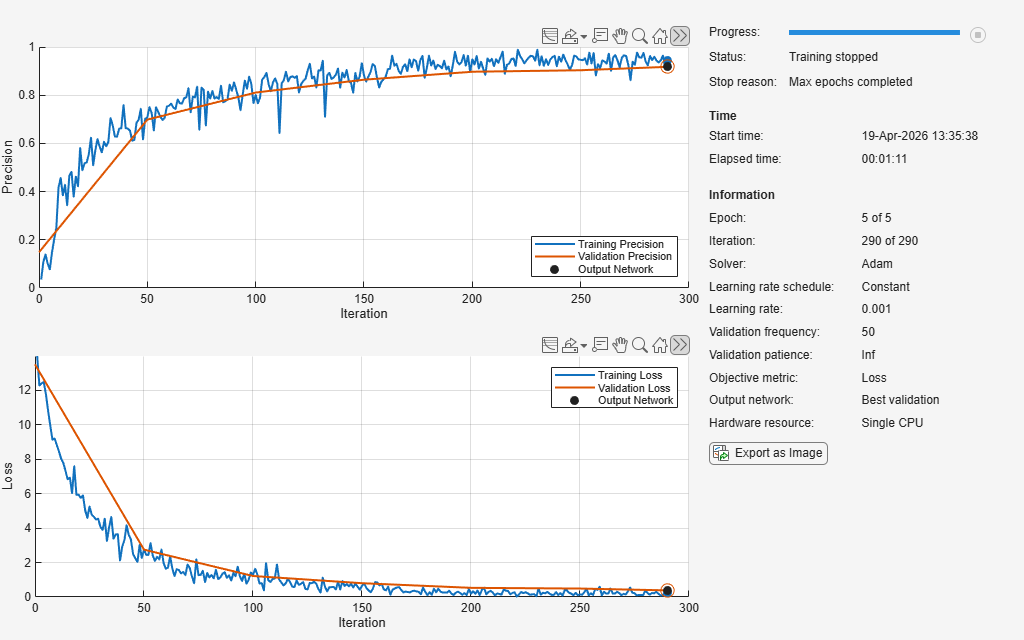# precisionMetric

Deep learning precision metric

Since R2023b

## Description

Use a `PrecisionMetric` object to track the precision when you train a deep neural network.

To specify which metrics to use during training, specify the `Metrics` option of the `trainingOptions` function. You can use this option only when you train a network using the `trainnet` function.

To plot the metrics during training, in the training options, specify `Plots` as `"training-progress"`. If you specify the `ValidationData` training option, then the software also plots and records the metric values for the validation data. To output the metric values to the Command Window during training, in the training options, set `Verbose` to `true`.

You can also access the metrics after training using the `TrainingHistory` and `ValidationHistory` fields from the second output of the `trainnet` function.

## Creation

### Syntax

``metric = precisionMetric``
``metric = precisionMetric(Name=Value)``

### Description

example

````metric = precisionMetric` creates a `PrecisionMetric` object. You can then specify `metric` as the `Metrics` name-value argument in the `trainingOptions` function. This metric is valid only for classification tasks.With no additional options specified, this syntax is equivalent to setting `Metrics="precision"` in the training options.```
````metric = precisionMetric(Name=Value)` sets the `Name`, `NetworkOutput`, `AverageType`, and `ClassificationMode` properties using name-value arguments.```

## Properties

expand all

Metric name, specified as a string scalar or character vector. The metric name appears in the training plot, the verbose output, and the training information that you can access as the second output of the `trainnet` function.

Data Types: `char` | `string`

Name of the output layer to apply the metric to, specified as `[]`, a string scalar, or a character vector. When the value is `[]`, the software passes all of the network outputs to the metric.

Note

You can apply the built-in metric to only a single output. If you have a network with multiple outputs, then you must specify the `NetworkOutput` name-value argument. To apply built-in metrics to multiple outputs, you must create a metric object for each output.

Data Types: `char` | `string`

Type of averaging to use to compute the metric, specified as one of these values:

• `"micro"` — Calculate the metric across all classes.

• `"macro"` — Calculate the metric for each class and return the average.

• `"weighted"` — Calculate the metric for each class and return the weighted average. The weight for a class is the proportion of observations from that class.

Data Types: `char` | `string`

Type of classification task, specified as one of these values:

• `"single-label"` — Each observation is exclusively assigned one class label (single-label classification).

• `"multilabel"` — Each observation can be assigned more than one independent class label (multilabel classification). The software uses a softmax threshold of 0.5 to assign class labels.

To select the classification mode for binary classification, consider the output layer of the network:

• If the final layer has an output size of one, such as with a sigmoid layer, use `"multilabel"`.

• If the final layer has an output size of two, such as with a softmax layer, use `"single-label"`.

Note

This metric is not supported when the `ClassificationMode` is set to `"single-label"` and the network output has a channel dimension of size 1. For example, if you have a single class and your output layer is a `sigmoidLayer` (binary-sigmoid task).

Data Types: `char` | `string`

## Object Functions

 `trainingOptions` Options for training deep learning neural network `trainnet` Train deep learning neural network

## Examples

collapse all

Plot and record the training and validation precision when you train a deep neural network.

Unzip the digit sample data and create an image datastore. The `imageDatastore` function automatically labels the images based on folder names.

```unzip("DigitsData.zip") imds = imageDatastore("DigitsData", ... IncludeSubfolders=true, ... LabelSource="foldernames");```

The datastore contains 10,000 synthetic images of digits from 0 to 9. Each image in the data set has a size of 28-by-28-by-1 pixels. You can train a deep learning network to classify the digit in the image.

Use a subset of the data as the validation set.

```numTrainingFiles = 750; [imdsTrain,imdsVal] = splitEachLabel(imds,numTrainingFiles,"randomize");```

Create an image classification network.

```layers = [ ... imageInputLayer([28 28 1]) convolution2dLayer(5,20) reluLayer maxPooling2dLayer(2,Stride=2) fullyConnectedLayer(10) softmaxLayer];```

Create a `PrecisionMetric` object and set `AverageType` to `"macro"` . You can use this object to record and plot the training and validation precision.

`metric = precisionMetric(AverageType="macro")`
```metric = PrecisionMetric with properties: Name: "Precision" AverageType: "macro" ClassificationMode: "single-label" NetworkOutput: [] ```

Specify the precision metric in the training options. To plot the precision during training, set `Plots` to `"training-progress"`. To output the values during training, set `Verbose` to `true`.

```options = trainingOptions("adam", ... MaxEpochs=5, ... Metrics=metric, ... ValidationData=imdsVal, ... ValidationFrequency=50, ... Plots="training-progress", ... Verbose=true);```

Train the network using the `trainnet` function.

`[net,info] = trainnet(imdsTrain,layers,"crossentropy",options);`
``` Iteration Epoch TimeElapsed LearnRate TrainingLoss ValidationLoss TrainingPrecision ValidationPrecision _________ _____ ___________ _________ ____________ ______________ _________________ ___________________ 0 0 00:00:03 0.001 13.488 0.14828 1 1 00:00:03 0.001 13.974 0.035 50 1 00:00:22 0.001 2.7427 2.7444 0.70805 0.6987 100 2 00:00:40 0.001 1.2925 1.2188 0.80145 0.81142 150 3 00:01:11 0.001 0.65133 0.79837 0.88205 0.86567 200 4 00:01:27 0.001 0.19458 0.5328 0.94937 0.89822 250 5 00:01:45 0.001 0.14988 0.4882 0.94726 0.90363 290 5 00:02:00 0.001 0.2756 0.40297 0.93726 0.91526 Training stopped: Max epochs completed ```Access the loss and precision values for the validation data.

`info.ValidationHistory`
```ans=7×3 table Iteration Loss Precision _________ _______ _________ 0 13.488 0.14828 50 2.7444 0.6987 100 1.2188 0.81142 150 0.79837 0.86567 200 0.5328 0.89822 250 0.4882 0.90363 290 0.40297 0.91526 ```